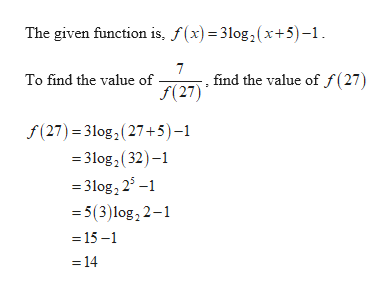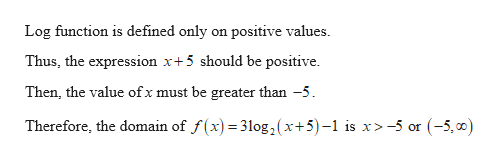# Suppose we have the logarithmic function f(x)= 3log2(x+5)-1.a.) Simplify: 7/f(27)b.) What's the domain of f?c.) Solve the following equation: f(2x)= -4

Question
9 views

Suppose we have the logarithmic function f(x)= 3log2(x+5)-1.

a.) Simplify: 7/f(27)

b.) What's the domain of f?

c.) Solve the following equation: f(2x)= -4

check_circle

Step 1help_outlineImage TranscriptioncloseThe given function is, f(x)= 3log,2(x+5)-1 . find the value of f(27) f(27) To find the value of f(27) 3log2(27+5) -1 =31og2(32- =3log, 25- 5(3)log, 2-1 = 15-1 14 fullscreen
Step 2help_outlineImage TranscriptioncloseLog function is defined only on positive values. Thus, the expression x+5 should be positive Then, the value ofx must be greater than -5 Therefore, the domain of f(x) 31log , ( x+5)-1 is x>-5 or (-5,0) fullscreen

### Want to see the full answer?

See Solution

#### Want to see this answer and more?

Solutions are written by subject experts who are available 24/7. Questions are typically answered within 1 hour.*

See Solution
*Response times may vary by subject and question.
Tagged in

### Logarithms